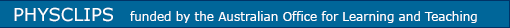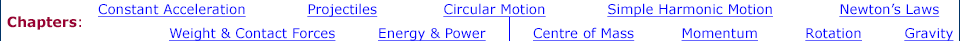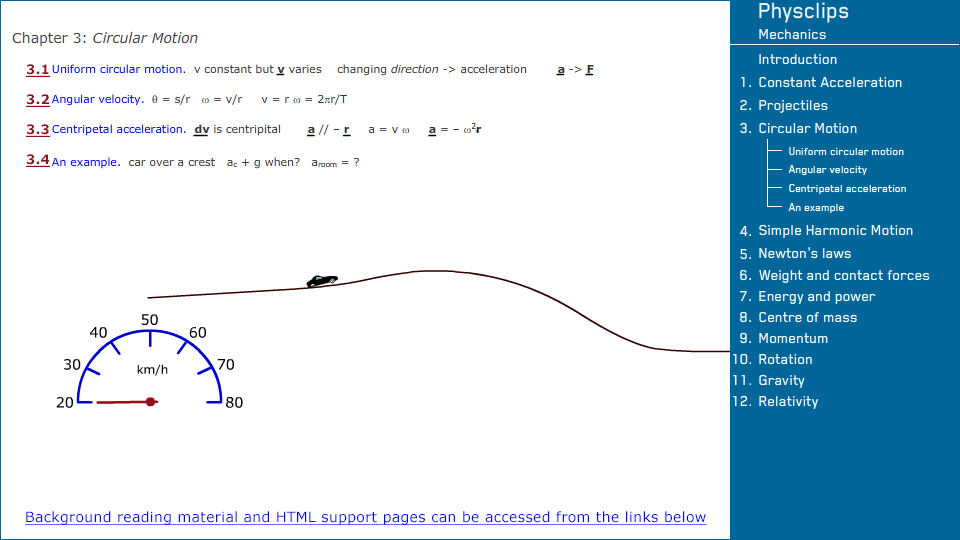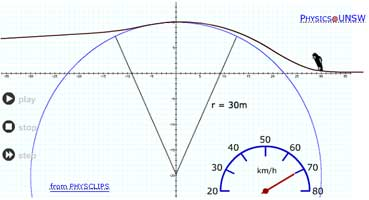Links to related material and teachers' resources Background material for circular motion Because the direction changes in circular motion, the velocity changes, even if the speed is constant. Simple vector arguments give the centripetal acceleration in terms of radius and speed or radius and angular velocity. Several examples follow. Rolling: the physics of wheels Vectors Vectors, vector components, unit vectors, two and three dimensions, vector addition and subtraction, relative motion and moving coordinates, the scalar product of vectors (the dot product), the vector product (the cross product) A simple introduction to calculus: Differentials and integrals A simple, physics-based guide to quantities that vary: differentiation and integration, numerical and analytical, the log function, polynomials, trigonometric functions, the chain rule, techniques for integration, partial derivatives, introduction to logs Download all animations in this chapter ( mp4 videos / original flash files ) Or you can download individually below each thumbnail Circular motionDownload (mp4 video)Download (original flash file)